# Excel 破解工作表密碼，免額外下載 App 全版本適用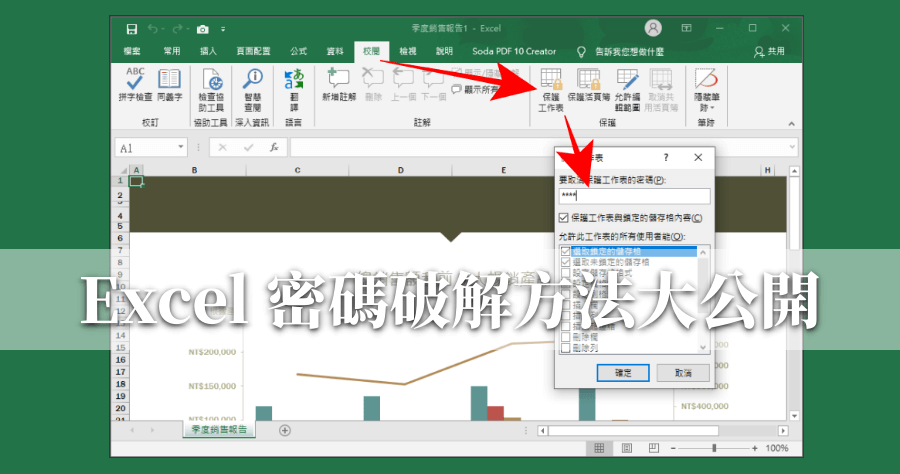Excel 密碼保護的工作表，如何破解密碼呢？你有沒有曾經打開一個陳年 Excel 檔案，是自己曾經加密的，如果要編輯則需要解除密碼才能編輯？但早就已經不知道密碼是多少了呢～今天要跟大家分享一個方法，不需要透過任何 APP，只需要幾個步驟，就可以解除 Excel 工作表的密碼，趕快來跟小編一起看看如何破解吧！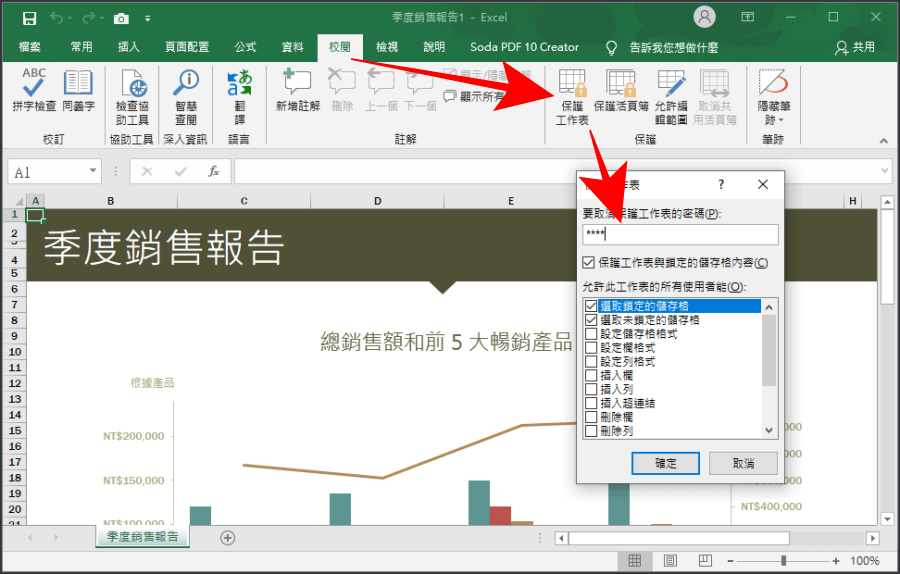△ 在破解密碼之前，小編就先建立一個具有密碼保護的 Excel 工作表，上方「校閱」>「保護工作表」>「輸入密碼」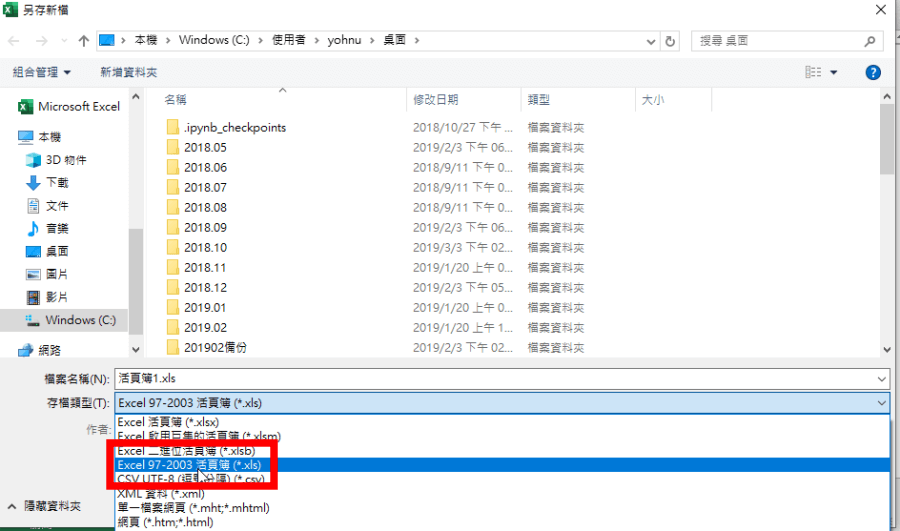△ 今天要跟大家分享的方法，適合所有 Excel 版本。小編的 Excel 版本是 2019 年，設好密碼之後，將 Excel 檔案另存新檔，並在下方的存檔類型選擇「97-2003 活頁簿」的 xls 檔。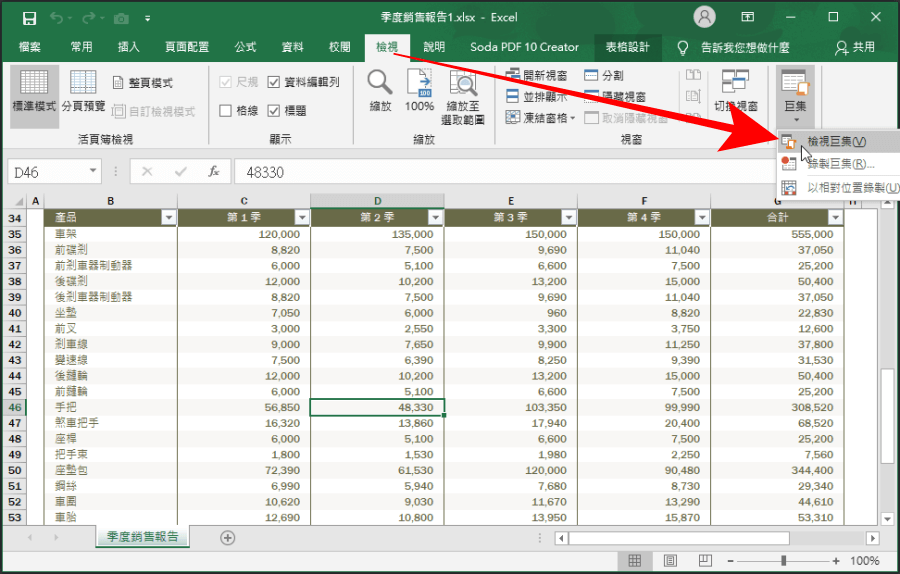△ 儲存好檔案之後，開啟儲存的新檔案，這時候要建立一個破解密碼的巨集，找到上方「檢視」>「巨集」>「檢視巨集」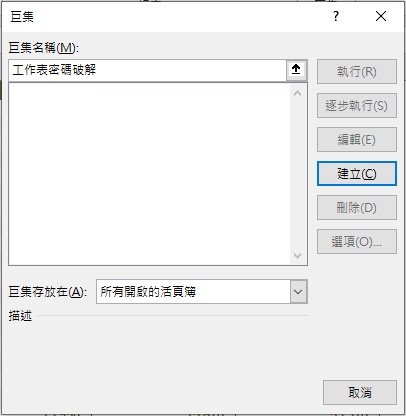△ 在「巨集名稱」任意輸入名稱，然後按下「建立」。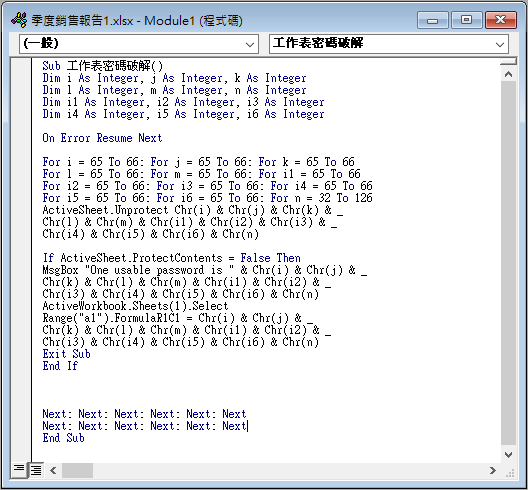△ 接著在第一段「Sub 工作表密碼破解」及最後一段「End Sub」中間加入以下代碼，完成後如上圖所示：

Dim i As Integer, j As Integer, k As Integer
Dim l As Integer, m As Integer, n As Integer
Dim i1 As Integer, i2 As Integer, i3 As Integer
Dim i4 As Integer, i5 As Integer, i6 As Integer

On Error Resume Next

For i = 65 To 66: For j = 65 To 66: For k = 65 To 66
For l = 65 To 66: For m = 65 To 66: For i1 = 65 To 66
For i2 = 65 To 66: For i3 = 65 To 66: For i4 = 65 To 66
For i5 = 65 To 66: For i6 = 65 To 66: For n = 32 To 126
ActiveSheet.Unprotect Chr(i) & Chr(j) & Chr(k) & _
Chr(l) & Chr(m) & Chr(i1) & Chr(i2) & Chr(i3) & _
Chr(i4) & Chr(i5) & Chr(i6) & Chr(n)

If ActiveSheet.ProtectContents = False Then
MsgBox “One usable password is ” & Chr(i) & Chr(j) & _
Chr(k) & Chr(l) & Chr(m) & Chr(i1) & Chr(i2) & _
Chr(i3) & Chr(i4) & Chr(i5) & Chr(i6) & Chr(n)
ActiveWorkbook.Sheets(1).Select
Range(“a1”).FormulaR1C1 = Chr(i) & Chr(j) & _
Chr(k) & Chr(l) & Chr(m) & Chr(i1) & Chr(i2) & _
Chr(i3) & Chr(i4) & Chr(i5) & Chr(i6) & Chr(n)
Exit Sub
End If

Next: Next: Next: Next: Next: Next
Next: Next: Next: Next: Next: Next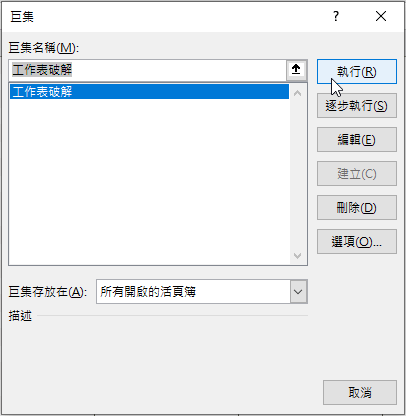△ 貼上後，回到原本的 Excel 工作表，找到最上方的「檢視」>「巨集」>「檢視巨集」，執行剛剛所新增的巨集。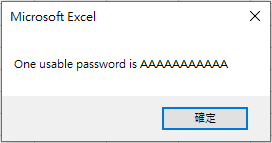△ 這時候看到上面的訊息，就代表 Excel 工作表密碼已經被破解啦，這時就可以編輯、重設密碼囉！以上方法分享給忘記 Excel 工作表密碼的朋友們，完全免 App 就能解除密碼，大家擔心忘記了話，趕快把此文章存在書籤中，忘記的時候就可以隨時回來查看囉！